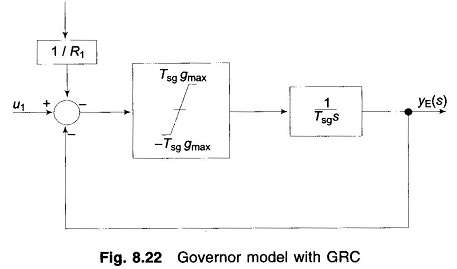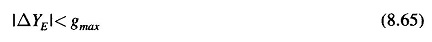## Load Frequency Control with Generation Rate Constraint (GRC):

Load Frequency Control with Generation Rate Constraint (GRC) – The Load frequency Control problem discussed so far does not consider the effect of the restrictions on the rate of change of power generation. In power systems having steam plants, power generation can change only at a specified maximum rate. The generation rate (from safety considerations of the equipment) for reheat units is quit low.

Most of the reheat units have a generation rate around 3%/min. Some have a generation rate between 5 to 10%/min If these constraints are not considered, system is likely to chase large momentary disturbances. This results in undue wear and tear of the controller.

Several methods have been proposed to consider the effect of GRCs for the design of automatic generation controllers. When Generation Rate Constraint is considered, the system dynamic model becomes non-linear and linear control techniques cannot be applied for the optimization of the controller setting.

If the generation rates denoted by PGi are included in the state vector, the system order will be altered. Instead of augmenting them, while solving the state equations, it may be verified at each step if the GRCs are violated.Another way of considering Generation Rate Constraint for both areas is to add limiters to the governors as shown in Fig. 8.22, i.e., the maximum rate of valve opening or closing speed is restricted by the limiters. Here Tsg gmax is the power rate limit imposed by valve or gate control. In this modelThe banded values imposed by the limiters are selected to restrict the generation rate by 10% per minute.

The Generation Rate Constraint result in larger deviations in ACEs as the rate at which generation can change in the area is constrained by the limits imposed. Therefore, the duration for which the power needs to be imported increases considerably as compared to the case where generation rate is not constrained. With GRCs, R should be selected with care so as to give the best dynamic response.

In hydro-thermal system, the generation rate in the hydro area normally remains below the safe limit and therefore GRCs for all the hydro plants can be ignored.

Scroll to Top